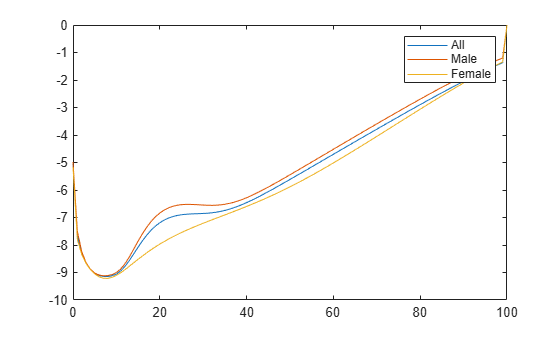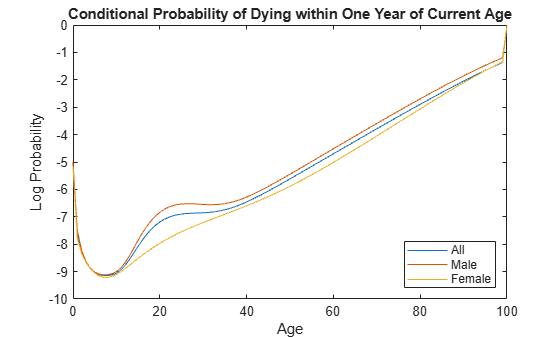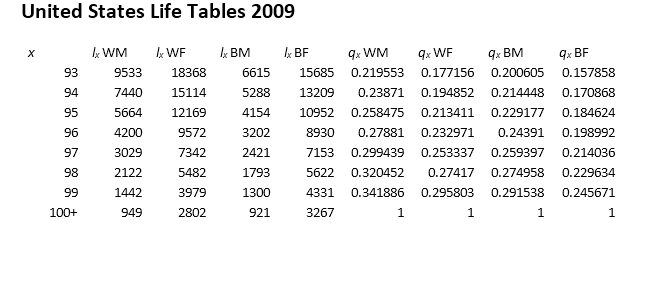# lifetablegen

Generate life table series from calibrated mortality model

## Syntax

``````[qx,lx,dx] = lifetablegen(x,a)``````
``````[qx,lx,dx] = lifetablegen(x,a,lifemodel)``````

## Description

example

``````[qx,lx,dx] = lifetablegen(x,a)``` generates a life table series from a calibrated mortality model.```

example

``````[qx,lx,dx] = lifetablegen(x,a,lifemodel)``` generates a life table series from a calibrated mortality model using the optional argument for `lifemodel`.```

## Examples

collapse all

Load the life table data file.

`load us_lifetable_2009`

Calibrate the life table from survival data using the default `heligman-pollard` parametric model.

`a = lifetablefit(x, lx)`
```a = 8×3 0.0005 0.0006 0.0004 0.0592 0.0819 0.0192 0.1452 0.1626 0.1048 0.0007 0.0011 0.0007 6.2843 6.7637 1.1038 24.1387 24.2895 53.1783 0.0000 0.0000 0.0000 1.0971 1.0987 1.1100 ```

Generate a life table series from the calibrated mortality model.

```qx = lifetablegen(x,a); display(qx(1:20,:))```
``` 0.0063 0.0069 0.0057 0.0005 0.0006 0.0004 0.0002 0.0003 0.0002 0.0002 0.0002 0.0002 0.0001 0.0001 0.0001 0.0001 0.0001 0.0001 0.0001 0.0001 0.0001 0.0001 0.0001 0.0001 0.0001 0.0001 0.0001 0.0001 0.0001 0.0001 0.0001 0.0001 0.0001 0.0001 0.0001 0.0001 0.0002 0.0002 0.0001 0.0002 0.0002 0.0002 0.0002 0.0003 0.0002 0.0003 0.0004 0.0002 0.0004 0.0005 0.0002 0.0005 0.0006 0.0003 0.0006 0.0008 0.0003 0.0007 0.0009 0.0003 ```

Plot the `qx` series and display the legend. The series `qx` is the conditional probability that a person at age x will die between age x and the next age in the series.

```plot(x,log(qx)) legend(series)```Load the life table data file.

`load us_lifetable_2009`

Convert the life table series into life tables with forced termination.

`[~, lx] = lifetableconv(x, qx, 'qx');`

Calibrate the life table from survival data using the default `heligman-pollard` parametric model.

`a = lifetablefit(x, lx)`
```a = 8×3 0.0005 0.0006 0.0004 0.0592 0.0819 0.0192 0.1452 0.1626 0.1048 0.0007 0.0011 0.0007 6.2847 6.7634 1.1036 24.1385 24.2898 53.1918 0.0000 0.0000 0.0000 1.0971 1.0987 1.1100 ```

Generate a life table series from the calibrated mortality model.

`qx = lifetablegen((0:100), a)`
```qx = 101×3 0.0063 0.0069 0.0057 0.0005 0.0006 0.0004 0.0002 0.0003 0.0002 0.0002 0.0002 0.0002 0.0001 0.0001 0.0001 0.0001 0.0001 0.0001 0.0001 0.0001 0.0001 0.0001 0.0001 0.0001 0.0001 0.0001 0.0001 0.0001 0.0001 0.0001 ⋮ ```

Plot the `qx` series and display the legend. The series `qx` is the conditional probability that a person at age x will die between age x and the next age in the series.

```plot((0:100), log(qx)); legend(series, 'location', 'southeast'); title('Conditional Probability of Dying within One Year of Current Age'); xlabel('Age'); ylabel('Log Probability');```## Input Arguments

collapse all

Increasing ages for raw data, specified as a `N` vector of nonnegative integer values. The ages must start at `0` (birth).

Data Types: `double`

Model parameters for `num` models, specified as a `numparam`-by-`num` matrix, where the number of parameters (`numparam`) depends on the model specified using the `lifemodel` argument.

Data Types: `double`

(Optional) Parametric mortality model type, specified as a character vector with one of the following values:

• `'heligman-pollard'` — Eight-parameter Heligman-Pollard model (version 1), specified in terms of the discrete hazard function:

`$\frac{q\left(x\right)}{1-q\left(x\right)}={A}^{{\left(x+B\right)}^{C}}+D\mathrm{exp}\left(-E{\left(\mathrm{log}\frac{x}{F}\right)}^{2}\right)+G{H}^{X}$`

for ages x`0`, with parameters A, B, C, D, E, F, G, H`0`.

• `'heligman-pollard-2'` — Eight-parameter Heligman-Pollard model (version 2), specified in terms of the discrete hazard function:

`$\frac{q\left(x\right)}{1-q\left(x\right)}={A}^{{\left(x+B\right)}^{C}}+D\mathrm{exp}\left(-E{\left(\mathrm{log}\frac{x}{F}\right)}^{2}\right)+\frac{G{H}^{X}}{1+G{H}^{X}}$`

for ages x`0`, with parameters A, B, C, D, E, F, G, H`0`.

• `'heligman-pollard-3'` — Eight-parameter Heligman-Pollard model (version 3), specified in terms of the discrete hazard function:

`$q\left(x\right)={A}^{{\left(x+B\right)}^{C}}+D\mathrm{exp}\left(-E{\left(\mathrm{log}\frac{x}{F}\right)}^{2}\right)+G{H}^{X}$`

for ages x`0`, with parameters A, B, C, D, E, F, G, H`0`.

• `'gompertz'` — Two-parameter Gompertz model, specified in terms of the continuous hazard function:

`h(x) = A exp(Bx)`
for ages x`0`, with parameters A, B`0`.

• `'makeham'` — Three-parameter Gompertz-Makeham model, specified in terms of the continuous hazard function:

`h(x) = A exp(Bx) + C`
for ages x`0`, with parametersA, B, C`0`.

• `'siler'` — Five-parameter Siler model, specified in terms of the continuous hazard function:

`h(x) = A exp(Bx) + C + D exp(-Ex)`
for ages x`0`, with parameters A, B, C, D, E`0`.

Data Types: `char`

## Output Arguments

collapse all

Conditional probabilities of dying for `N` ages and `num` series, returned as an `N`-by-`num` matrix. The series `qx` is the conditional probability that a person at age x will die between age x and the next age in the series. For the last age, `qx` represents probabilities or counts for all ages after the last age.

The last row of the `N`-by-`num` output for `qx` is the values for all ages on or after the last age in x (due to Forced Termination). Therefore, the last row of `qx` contains `1` (100% probability of dying on or after the last age).

Survival counts for `N` ages and `num` series, returned as an `N`-by-`num` matrix. The series `lx` is the number of people alive at age x, given 100,000 alive at birth.

Decrement counts for `N` ages and `num` series, returned as an `N`-by-`num` matrix. The series `dx` is the number of people out of 100,000 alive at birth who die between age `x` and the next age in the series. For the last age, `dx` represent probabilities or counts for all ages after the last age.

The last row of the `N`-by-`num` output for `dx` are values for all ages on or after the last age in x (due to Forced Termination). Therefore, the last row of `dx` contains the remaining count of 100,000 people alive at birth who have not died by the last age.

collapse all

### Forced Termination

Most modern life tables have “forced” termination. Forced termination means that the last row of the life table applies for all persons with ages on or after the last age in the life table.

This sample illustrates forced termination.In this case, the last row of the life table applies for all persons aged 100 or older. Specifically, qx probabilities are 1qx for ages less than 100 and, technically, qx for age 100.

Forced termination has terminal age values that apply to all ages after the terminal age so that `lx` is positive, `qx` is `1`, and `dx` is positive. Ages after the terminal age are `NaN` values, although `lx` and `dx` can be `0` and `qx` can be `1` for input series. Forced termination is triggered by a naturally terminating series, the last age in a truncated series, or the first `NaN` value in a series.

 Arias, E. “United States Life Tables.” National Vital Statistics Reports, U.S. Department of Health and Human Services. Vol. 62, No. 7, 2009.

 Carriere, F. “Parametric Models for Life Tables.” Transactions of the Society of Actuaries. Vol. 44, 1992, pp. 77–99.

 Gompertz, B. “On the Nature of the Function Expressive of the Law of Human Mortality, and on a New Mode of Determining the Value of Life Contingencies.” Philosophical Transactions of the Royal Society. Vol. 115, 1825, pp. 513–582.

 Heligman, L. M. A., and J. H. Pollard. “The Age Pattern of Mortality.” Journal of the Institute of Actuaries Vol. 107, Pt. 1, 1980, pp. 49–80.

 Makeham, W. M. “On the Law of Mortality and the Construction of Annuity Tables.” Journal of the Institute of Actuaries Vol. 8, 1860, pp. 301–310.

 Siler, W. “A Competing-Risk Model for Animal Mortality.” Ecology Vol. 60, pp. 750–757, 1979.

 Siler, W. “Parameters of Mortality in Human Populations with Widely Varying Life Spans.” Statistics in Medicine Vol. 2, 1983, pp. 373–380.